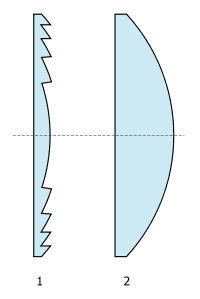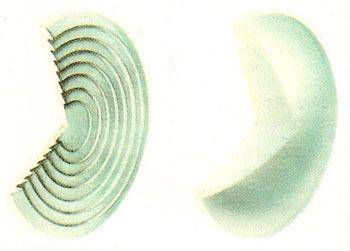# Harnessing Solar Energy with Fresnel Lenses

• Pranav Jha

#### Pranav Jha

i am about to do a project on harnessing solar energy using fresnel lens. However, i do not understand how a fresnel lens works. Could someone please clarify how it works (how can it converge light despite having a flat face) and why does it have more converging ability than other lenses?

i am about to do a project on harnessing solar energy using fresnel lens. However, i do not understand how a fresnel lens works. Could someone please clarify how it works (how can it converge light despite having a flat face) and why does it have more converging ability than other lenses?

I don't know about converging ability but this looks like a good starting point:
http://en.wikipedia.org/wiki/Fresnel_lens

I don't know about converging ability but this looks like a good starting point:
http://en.wikipedia.org/wiki/Fresnel_lens

Been through the article.
"The Fresnel lens reduces the amount of material required compared to a conventional spherical lens by dividing the lens into a set of concentric annular sections known as "Fresnel zones", which are theoretically limitless"
I didn't understand the above quoted statement.

I don't know about converging ability but this looks like a good starting point:
http://en.wikipedia.org/wiki/Fresnel_lens
In particular, I think this image from the Wiki article says it all:The two lenses in the figure have the same focusing power. Also, note the Fresnel lens is not perfectly flat.

Been through the article.
"The Fresnel lens reduces the amount of material required compared to a conventional spherical lens by dividing the lens into a set of concentric annular sections known as "Fresnel zones", which are theoretically limitless"
I didn't understand the above quoted statement.

I admit I don't understand the "theoretically limitless" part, but here is another view of a Fresnel lens (on left) showing the concentric circular zones:(From http://www.daviddarling.info/encyclopedia/F/AE_Fresnel_lens.html )

I admit I don't understand the "theoretically limitless" part, but here is another view of a Fresnel lens (on left) showing the concentric circular zone
(From http://www.daviddarling.info/encyclopedia/F/AE_Fresnel_lens.html )

The text there may have the answer to the other question of the OP: "allowing mechanically robust lenses of relatively short focal length to be fitted " - thus for big lenses a short focal length implies a weight problem, because they are thicker. The Fresnel lens does not have that drawback.

re "theoretically limitless part"
Ideally,for image formation,the emergent rays would converge at smoothly increasing angles from the centre of the lens outwards but the stepped structure of the lens results in a stepped convergence resulting in something similar to spherical aberration.For example if there was parallel incidence then,seen in cross section,there would be a number of emergent parallel beams one from each annular section.By increasing the number of annular sections the width of each beam would reduce and the lens would approach the ideal case.I think there must be limits on how many annular sections can be made but these are probably imposed more by practical rather than theoretical difficulties.

The general principle of a Fresnel lens is as follows.

- Imagine a flat pane of glass centred on an optical axis and another point P, also located on the optical axis.
- The distance between any point on the glass pane (call it X) and the point P will depend on the axial height of X (i.e. the distance from X to the optical axis).
- This path difference corresponds to a phase difference.
- The phase will alternate between being positive and negative as X moves outward from the optical axis.
- We can thus define zones of positive and negative phase contributions to the glass pane. This is the origin of Fresnel zones.
- Now, we can make a lens by preventing light from going through every second Fresnel zone. This ensures that the phase contribution at point P will only be positive (or negative), resulting in a focusing effect being obtained when a plane wave is passed through the glass pane.
- There are alternate ways to achieve this effect, for example adjusting the thickness of the glass pane in different Fresnel zones will achieve a similar effect.
- The distance between the glass pane and the point P is determined by the width of the Fresnel zones. This is in contrast to ordinary lens where the focal length depends on the curvature of the lens surface.

Re: the "theoretically limitless" part on the wikipedia articles, I think, given the context, it is saying that there are an unlimited number of Fresnel zones. A real lens, however will only span a finite number of these zones.

Claude.

The general principle of a Fresnel lens is as follows.

- Imagine a flat pane of glass centred on an optical axis and another point P, also located on the optical axis.
- The distance between any point on the glass pane (call it X) and the point P will depend on the axial height of X (i.e. the distance from X to the optical axis).
- This path difference corresponds to a phase difference.
- The phase will alternate between being positive and negative as X moves outward from the optical axis.
- We can thus define zones of positive and negative phase contributions to the glass pane. This is the origin of Fresnel zones.
- Now, we can make a lens by preventing light from going through every second Fresnel zone. This ensures that the phase contribution at point P will only be positive (or negative), resulting in a focusing effect being obtained when a plane wave is passed through the glass pane.
- There are alternate ways to achieve this effect, for example adjusting the thickness of the glass pane in different Fresnel zones will achieve a similar effect.
- The distance between the glass pane and the point P is determined by the width of the Fresnel zones. This is in contrast to ordinary lens where the focal length depends on the curvature of the lens surface.

Re: the "theoretically limitless" part on the wikipedia articles, I think, given the context, it is saying that there are an unlimited number of Fresnel zones. A real lens, however will only span a finite number of these zones.

Claude.

Hi Claude,
Here you are describing zone plate lenses which are base on diffraction,the OP, however, was enquiring about Fresnel lenses which are based on refraction.The diagram posted by Redbelly shows such a lens in cross section and you will see that there is a smoothly varying convex section in the middle surrounded by a finite number of prismatic sections whose angles increase from the centre outwards.

Agreed, a Fresnel zone plate is different than a Fresnel lens, in just the way Dadface describes.